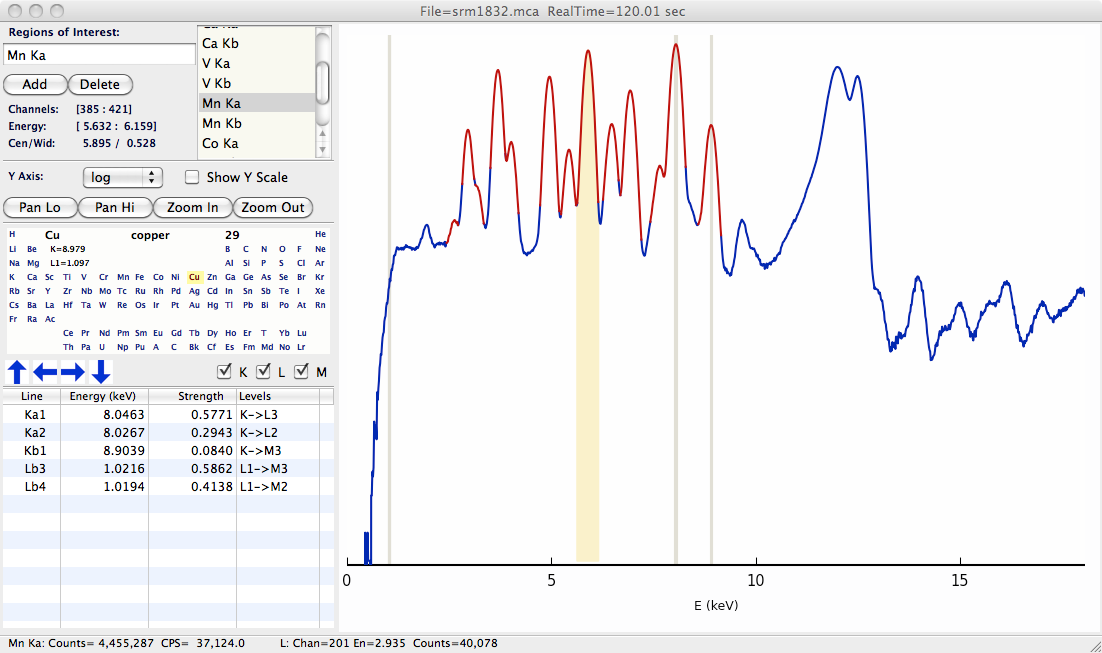# 15.1. XRF Data: MCA and ROI objects¶

XRF spectra are typically measured with MCAs (Multi Channel Analyzers) which holds an array of intensities. Because of the long history of using MCAs to store XRF spectra, the two concepts are often mixed, and one often talks about MCA traces as being the same as XRF spectra. To be sure, an XRF spectrum $$I(E)$$ can be represented well with an MCA trace (intensities per bin) as long as one can convert bin number to energy. Fortunately, most measurement systems in use have a linear relation (calibration) between bin number and energy, and the use of an MCA trace as an XRF spectrum is straightforward. We will probably use the terms interchangeably here as well.

Just to be clear: an XRF spectrum collected by an MCA will have a one dimensional array of counts with a single, well-defined energy for each bin. There will typically be between 1000 and 10,000 bins in each spectrum.

An ROI (Region of Interest) is a continuous portion of the XRF spectrum, generally representing a range of energies corresponding to a particular peak or X-ray emission line or family of lines. One often sums the counts in such an ROI to give a total number of counts for that emission line. An MCA spectrum may have many (typically 10s) of ROIs defined for particular emission lines.

In Larch, MCAs and ROIs are exposed as Groups, each with several attributes, and some built-in functions. For example, An MCA has arrays for energy and counts, as well as values for real_time and live_time, a deadtime correction factor dt_factor, and several ROIs. Each ROI has a left and right channel index, and a roi.get_counts() method.

## 15.1.1. Creating MCA objects¶

A simple way to create an MCA object is to read one from a disk file. For data collected at GSECARS, this can be done with the read_gsemca() function.

read a GSECARS MCA spectra file, returning a Group

Parameters

filename – name of GSECARS MCA file

The returned Group has the following components:

component name

description

filename

name of file

mcas

list of MCA objects for each MCA saved in the file

rois

list of ROIs

environ

list of Environmental Variables

energy

array of energy values

counts

array of counts, deadtime corrected and summed over MCAs

raw

array of counts, summed over MCAs, not corrected.

calib

dictionary of calibration values

dt_factor

real_time

real time for data acquisition

live_time

live time for data acquisition

nchans

get_roi_counts()

function to get counts for a named ROI

save_mcafile()

function to save MCA to file

xrf_plot(energy, counts, mca=None)

create an interactive window for displaying an X-ray Fluorescence spectra

Parameters
• energy – array of energy values

• counts – array of counts

• mca – MCA group (as read from read_gsemca()), containing ROI definitions, calibration values, and related data.

An example plot is shown belowFigure 15.1.1.1 Example XRF Display, showing X-ray Fluorescence spectra, defined ROIs (in red), and Periodic Table for showing predicted emission lines.¶